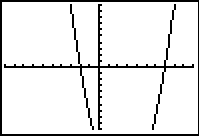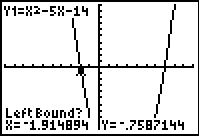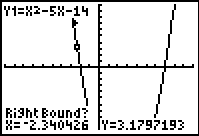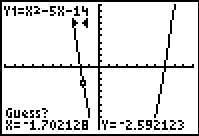Zeros of Functions

 The graphing calculator is very helpful when determining the zeros of functions (the locations were the graph crosses the x-axis --- the roots).

 Using the ZERO command: Solve: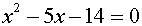Since this equation is set equal to zero, the zeros (roots) will be the locations where the graph crosses the x-axis (if the roots are real numbers). 1.  Set2.  Use the ZERO command to find the zeros (roots) --  2nd TRACE (CALC), #2 zero 3.  Left bound?  Move the spider as close to the zero (root --- where the graph crosses the x-axis) as possible.  Hit the left arrow to move to the "left" of the zero (root).  Hit ENTER.  A "marker" ► will be set to the left of the zero (root). 4.  Right bound?  Move the spider as close to the zero (root --- where the graph crosses the x-axis) as possible.  Hit the right arrow to move to the "right" of the zero (root).  Hit ENTER.  A "marker" ◄ will be set to the right of the zero (root). 5.  Guess?  Just hit ENTER. 6.  Repeat the entire process to find other zeros (roots --- which in this case the second root happens to be x = 7). Answer:  one of the zeros (roots) is x = -2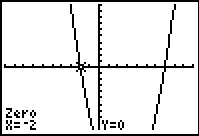Standard 10 x 10 window